# Banaschewski compactification

A topological spaceis-dimensional if it is a-space (cf. also Separation axiom) with a base of clopen sets (a set is called clopen if it is both open and closed). The Banaschewski compactification [a1], [a2] of, denoted by, is the-dimensional analogue of the Stone–Čech compactification of a Tikhonov space. It can be obtained as the Stone space of the Boolean algebra of clopen subsets.

The Banaschewski compactification is also a special case of the Wallman compactification [a4] (as generalized by N.A. Shanin, [a3]). A fairly general approach subsuming the above-mentioned compactifications is as follows.

Letbe an arbitrary non-empty set and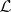a lattice of subsets ofsuch that. Assume thatis disjunctive and separating, let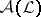be the algebra generated by, let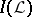be the set of non-trivial zero-one valued finitely additive measures on, and let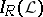be the set of elements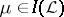that are-regular, i.e.,One can identifywith the-prime filters andwith the-ultrafilters (cf. also Filter; Ultrafilter).

Next, let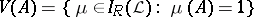, where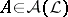;is a lattice isomorphism fromto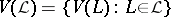. Take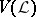as a base for the closed sets of a topology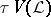on. Thenis a compact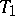-space and it is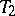(cf. Hausdorff space) if and only ifis a normal lattice.can be densely imbedded inby the mapping, whereis the Dirac measure concentrated at(cf. also Dirac delta-function). The mapping is a homeomorphism ifis given the topology of closed sets withas base for the closed sets.

Ifis a-space andis the lattice of closed sets, thenbecomes the usual Wallman compactification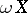.

Ifis a Tikhonov space andis the lattice of zero sets, then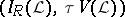becomes the Stone–Čech compactification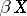.

Ifis a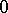-dimensional-space andis the lattice of clopen sets, thenbecomes the Banaschewski compactification.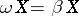if and only ifis a normal space;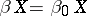if and only ifis strongly-dimensional (i.e., the clopen sets separate the zero sets).